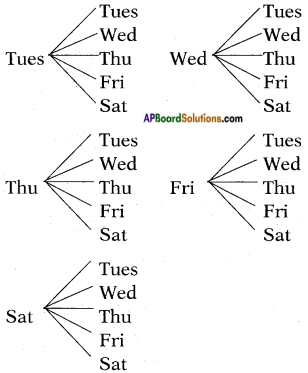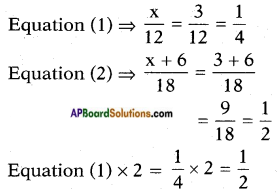## AP State Syllabus SSC 10th Class Maths Solutions 13th Lesson Probability Optional Exercise

AP State Board Syllabus AP SSC 10th Class Maths Textbook Solutions Chapter 13 Probability Optional Exercise Textbook Questions and Answers.

### 10th Class Maths 13th Lesson Probability Optional Exercise Textbook Questions and Answers

Question 1.
Two customers Shyam and Ekta are visiting a particular shop in the same week (Tuesday to Saturday). Each is equally likely to visit the shop on any day as on another day. What is the probability that both will visit the shop on
(i) the same day?
(ii) consecutive days?
(iii) different days?
Shyam and Ekta can visit the shop in the following combination:(T Tu) (W, Tu) (Th, Tu) (F, Tu) (S, Tu) (Tu, W) (W, W) (Th, W) (F, W) (S, W) (Tu, Th) (W, Th) (Th, F) (F, Th) (S, Th) (Tu, F) (W, F) (Th, S) (F, F) (S, F) (Tu, S) (W, S) (Th, Th) (F, S) (S, S)
∴ Number of total outcomes = 5 × 5 = 52 = 25 [also from the above table]
i) Number of favourable outcomes to that of visiting on the same day
(Tu, Tu), (W, W), (Th, Th), (F, F), (S, S) = 5
∴ Probability of visiting the shop on the same day = $$\frac{\text { No. of favourable outcomes }}{\text { No. of total outcomes }}$$ = $$\frac{5}{25}$$ = $$\frac{1}{5}$$
ii) Number of outcomes favourable to consecutive days
(Tu, W), (W, Th), (Th, F), (F, S), (W, Tu), (Th, W), (F, Th), (S, F) = 8
∴ Probability of visiting the shop on consecutive days = $$\frac{8}{25}$$
iii) If P(E) is the probability of visiting the shop on the same day,
then P($$\overline{\mathrm{E}}$$) is the probability of visiting the shop not on the same day.
i.e., P($$\overline{\mathrm{E}}$$) is the probability of visiting the shop on different days.
Such that P(E) + P($$\overline{\mathrm{E}}$$) = 1
P($$\overline{\mathrm{E}}$$) = 1 – P(E) = 1 – $$\frac{1}{5}$$ = $$\frac{4}{5}$$Question 2.
A bag contains 5 red balls and some blue balls. If the probability of drawing a blue ball is double that of a red ball, determine the number of blue balls in the bag.
Number of red balls in the bag = 5 As the probability of blue balls is double the probability of red balls, we have that number of blue balls is double the number of red balls.
∴ Blue balls = 5 × 2 = 10.
[!! Let the number of blue balls = x
Number of red balls = 5
Total no. of balls = x + 5
Total outcomes in drawing a ball at random = x + 5
Number of outcomes favourable to red ball = 5
∴ P(R) = $$\frac{5}{x+5}$$
from the problem,
P(B) = 2 × $$\frac{5}{x+5}$$ = $$\frac{10}{x+5}$$
Also $$\frac{5}{x+5}$$ + $$\frac{10}{x+5}$$ = 1
[∵ P(E) + P($$\overline{\mathrm{E}}$$) = 1]
⇒ $$\frac{5+10}{x+5}$$ = 1
⇒ $$\frac{15}{x+5}$$ = 1
⇒ x + 5 = 15]

Question 3.
A box contains 12 balls out of which x are black. If one ball is drawn at random from the box, what is the probability that it will be a black ball? If 6 more black balls are put in the box, the probability of drawing a black ball is now double of what it was before. Find x.
Number of black balls = x
Total number of balls in the box = 12
Probability of drawing a black ball = $$\frac{\text { No. of favourable outcomes }}{\text { No. of total outcomes }}$$ = $$\frac{x}{12}$$ …….. (1)
When 6 more black balls are placed in the box, number of favourable outcomes to black ball becomes = x + 6.
Total number of balls in the box becomes = 12 + 6 = 18.
Now the probability of drawing a black ball become = $$\frac{x+6}{18}$$ …….. (1)
By Problem,
$$\frac{x+6}{18}$$ = 2. $$\frac{x}{12}$$
⇒ $$\frac{x+6}{18}$$ = $$\frac{x}{6}$$
⇒ 6(x + 6) = 18(x)
⇒ 6x + 36 = 18x
⇒ 18x – 6x = 36
⇒ 12x = 36
⇒ x = $$\frac{36}{12}$$ = 3
Check:and hence proved.Question 4.
A jar contains 24 marbles, some are green and others are blue. If a marble is drawn at random from the jar, the probability that it is green is 2/3. Find the number of blue marbles in the jar.
Total number of marbles in the jar = 24.
Let the number of green marbles = x.
Then number of blue marbles = 24 – x.
Probability of drawing a green marbles = $$\frac{\text { No. of favourable outcomes }}{\text { No. of total outcomes }}$$ = $$\frac{x}{24}$$
By problem,
$$\frac{x}{24}$$ = $$\frac{2}{3}$$
⇒ 3x = 24 × 2
x = $$\frac{24 \times 2}{3}$$ = 16
∴ Number of green marbles = 16
Number of blue marbles = 24 – 1 = 8
∴ Probability of picking blue marble = $$\frac{8}{24}$$ = $$\frac{1}{3}$$
(OR)
P(B) = P(E) – P(G) = 1 – $$\frac{2}{3}$$ = $$\frac{1}{3}$$
[!! P(G) = $$\frac{2}{3}$$
P(G) + P(B) = 1
∴ P(B) = 1 – P(G) = 1 – $$\frac{2}{3}$$ = $$\frac{1}{3}$$
Number of blue marbles in the jar = $$\frac{1}{3}$$ × 24 = 8]